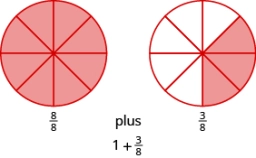# Work out

Work out the sum of 1/5, 3/4, and 1/2. Give your answer as a mixed number in its simplest form.

x =  1 920 = 29/20

### Step-by-step explanation:Did you find an error or inaccuracy? Feel free to write us. Thank you!

Tips for related online calculators
Need help calculating sum, simplifying, or multiplying fractions? Try our fraction calculator.
Need help with mixed numbers? Try our mixed-number calculator.